#### Study Material and Notes of Ch 11 Force and Pressure Class 8th Science

Topics in the chapter

• Introduction
• Force
→ Push
→ Pull
• Muscular Force
• Friction
• Magnetic Force
• Electrostatic Force
• Gravitational Force
• Pressure
• Fluid
• Atmospheric Pressure

Introduction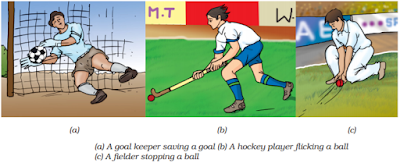→ Actions like picking, opening, shutting, kicking, hitting, lifting, flicking, pushing, pulling are often used to describe certain tasks.

→ Each of these actions usually results in some kind of change in the motion of an object.

Force

→ A push or pull on an object is called a force.• Push: When an object is moving away from the applier of force.

• Pull: When an object is moving towards the applier of force.

→ Force is a push or a pull which changes or tends to change the state of rest or of uniform motion, or direction of motion or the shape or size of a body.

→ Force is any action that has the tendency to change the position, shape, or size of an object.

→ Interaction of one object with another object results in a force between the two objects.

→ The effect of force depends on the magnitude and direction of the force.

→ Force applied in the same direction added to one another.

→ Force applied in the opposite direction, the net force is given by the difference of two forces.

→ Force can move a body initially at rest.

→ Force can bring a moving body to rest.

→ Force can change the direction of a moving body.

→ Force can change the speed of a moving body.

→Force can change the shape of a body.

→ Force can change the size of a body.

Muscular force→ It involves the action of muscles.

→ Animals make use of muscular force to carry out their physical activities and other tasks.

Friction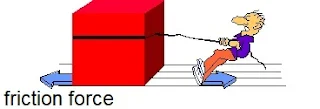→ It is an opposing force that acts between surfaces in contact moving with respect to each other.

→ The direction of force of friction is always opposite to the direction of motion.

→ Frictional force always acts between two moving objects, which are in contact with one another.

→ Frictional force always acts opposite to the direction of motion.

→ Frictional force depends on the nature of the surface in contact.

→ Non-contact force come into play even when the bodies are not in contact.

Magnetic force→ Force acting between two magnets or a magnet and a magnetic material.
Example: iron, steel, nickel, cobalt etc.).

→ It can be attractive and repulsive.

Electrostatic force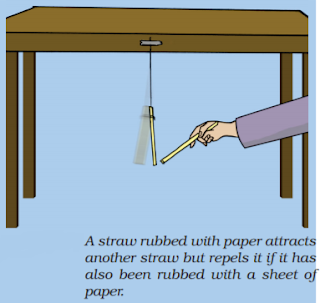→ Force due to electric charges.

→ It can be attractive and repulsive.

Gravitational force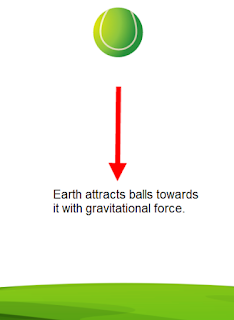→ It is a kind of attractive force that comes into play because of the mass of a body.
Example: earth's gravitational attraction.

Pressure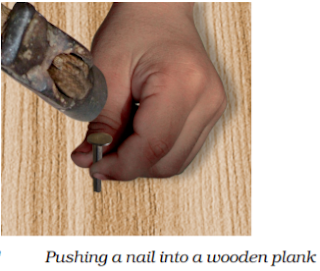→ The force acting per unit area of surface is called pressure.

• Pressure = Force/Area on which it acts

→ The unit of pressure is Newton per square meter (N/m2), which is also known as Pascal.

→ Smaller the area larger the pressure for the same force.

→ Liquids exerts pressure on the walls of the container.

→ Pressure exerted by liquids increases with depth.

→ Liquids exert equal pressure at the same depth.

→ The pressure at which water comes out of the holes is directly proportional to its depth.

Fluid

→ Substance which can flow and has no fixed shape

• Pressure due to a liquid column of height h (p) = hrg

where, h = Height of column
r = Density of fluid
g = Acceleration due to gravity

→ Pressure inside a fluid increases with increase in depth and density of the fluid.

→  Water and gas exert pressure on the walls of their container.

→ Atmosphere exerts pressure on the surface of the Earth.• Atmospheric pressure = Weight of the atmosphere per unit area.

Pressure inside our body is equal to the atmospheric pressure and cancels the pressure from out side.

Air surrounding the Earth: atmosphere

Air exerts pressure on its surroundings: thrust on unit area is called atmospheric pressure